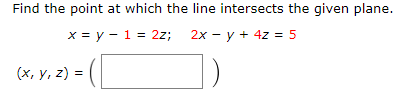Home / Answered Questions / Other / find-the-point-at-which-the-line-intersects-the-given-plane-x-y-1-2z-2x-y-4z-5-x-y-z-aw416

# (Solved): Find The Point At Which The Line Intersects The Given Plane. X = Y - 1 = 2z; 2x - Y + 4z = 5 (x, Y, ...Find the point at which the line intersects the given plane. x = y - 1 = 2z; 2x - y + 4z = 5 (x, y, z) = ( )

We have an Answer from Expert